The Rocscience International Conference 2021 Proceedings are now available. Read Now

# 15 - Back Analysis of Bolt Property

This tutorial will demonstrate how to perform back analysis of material properties using sensitivity analysis or probabilistic analysis features in SWedge.

Topics Covered in this Tutorial:

• Sensitivity Analysis
• Probabilistic Analysis
• Back Analysis of Bolt Properties

Finished Product:

The finished product of this tutorial can be found in the Tutorial 15 Back Analysis of Bolt Property.swd7 file, located in the Examples > Tutorials folder in your SWedge installation folder.

## 1.0 Introduction

Back analysis can be used to determine the required bolt strength to achieve a given safety factor. Sensitivity analysis can be used for back analysis of individual variables. Probabilistic analysis can be used for back analysis of multiple variables.

## 2.0 Model

If you have not already done so, run the SWedge program by double-clicking the SWedge icon in your installation folder or by selecting Programs > Rocscience > SWedge > SWedge in the Windows Start menu.

Let's start by opening the file from Tutorial 15 - Back Analysis of Bolt Property initial.swd7 in the Examples > Tutorials folder:Select: File > Open

You should see the following model.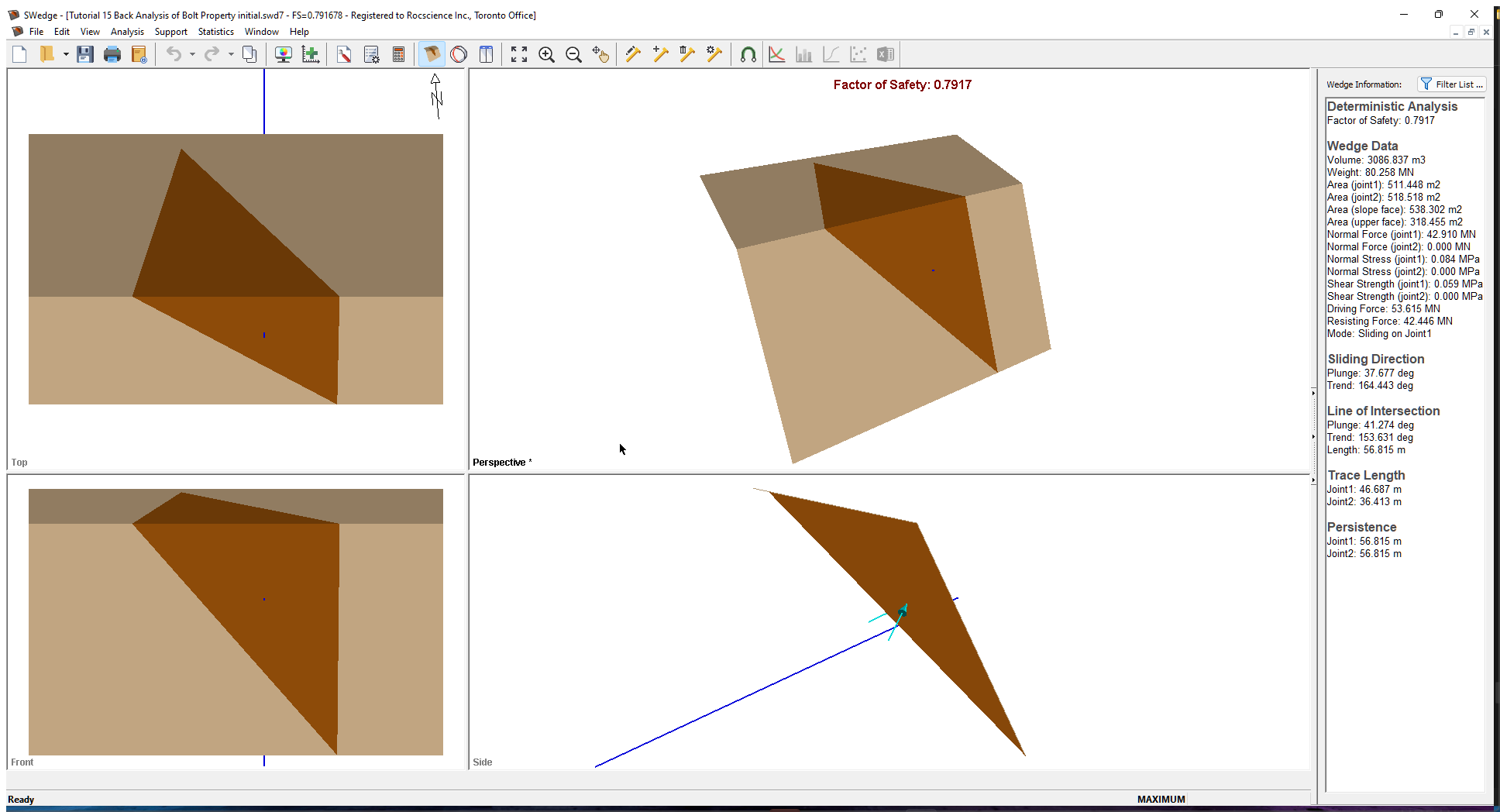The current slope is failing with a bolt of 30 MN/m capacity.

## 3.0 Sensitivity Analysis

Variations in the variables for a Sensitivity analysis are set up in the Sensitivity Input dialog. To open the dialog, select Sensitivity Analysis on the toolbar or Analysis menu.

1. Select Sensitivity Analysison the toolbar or Sensitivity on the Analysis menu.
2. Check the first check box in the dialog, select Passive Bolt Force from the dropdown list, and enter From = 20 and To = 60.3. Click OK..

The following graph is generated.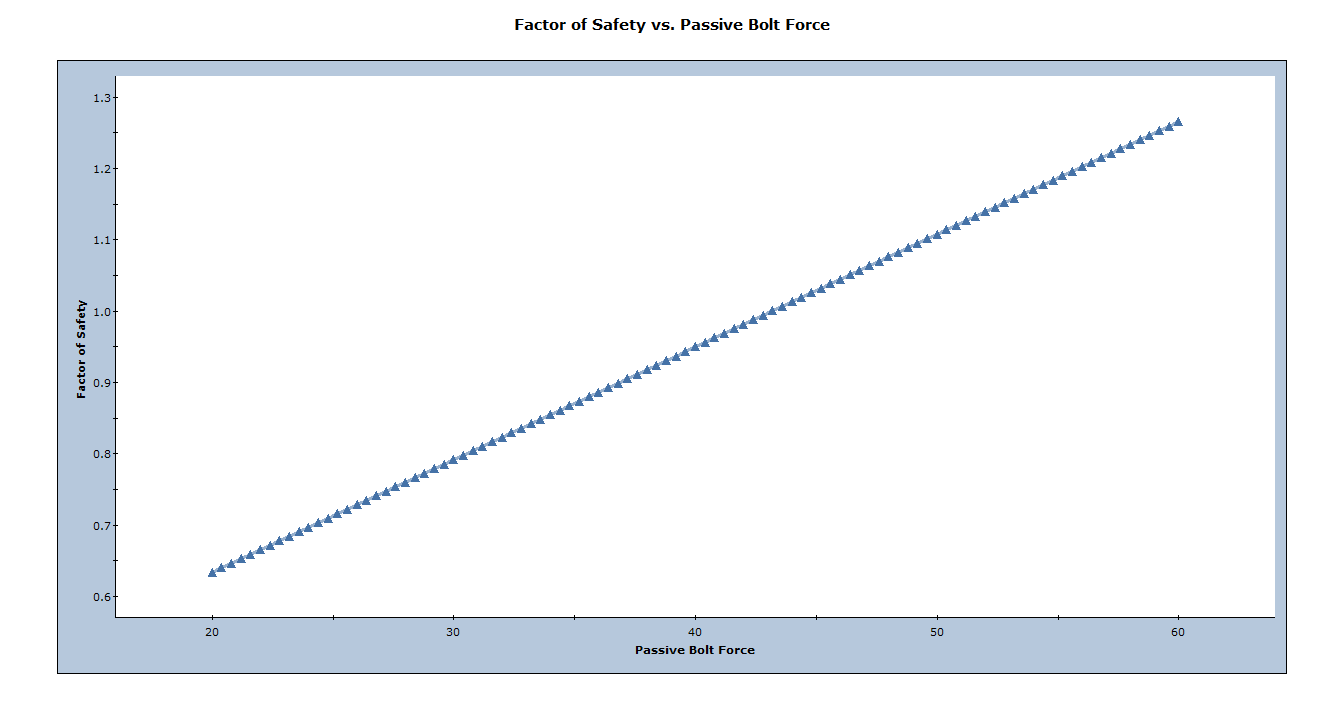We want to know the value of active bolt force which corresponds to safety factor = 1. We can do this as follows. Right-click on the plot and select Sampler > Edit Sampler. Two dotted crossing lines should appear. Scroll along the plot line until you obtain a factor of safety as close to 1.0 as possible. The cursor should snap along the plot line.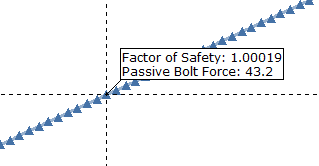We see that a value of 43.2 MN/m active bolt force corresponds to safety factor = 1.0. This assumes that all other slope parameters are exactly known and equal to their mean values.

To summarize: sensitivity analysis can easily be used for back analysis of individual variables. Just remember, when you are viewing a sensitivity plot of an individual variable, all other analysis variables are assumed to be constant and equal to their mean value. In general, this procedure may require some trial and error, for example if the desired safety factor is not within the output range of the sensitivity plot, you will have to adjust the allowable range of values of the variable and re-run the analysis.

Note: The Active/Passive Bolt Force in sensitivity analysis represents the sum of all the active/passive bolt forces in the model. If you have more than one bolt of the same bolt force model assigned, the active/passive bolt force will be the sum of all the bolts of the same bolt force model.

## 4.0 Probabilistic Analysis

### 4.1 PROJECT SETTINGS

To run a probabilistic analysis, we need to first change the analysis type to probabilistic in the Project Settings dialog. To open the dialog, select Project Settings on the toolbar or on the Analysis menu.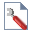Select: Analysis > Project Settings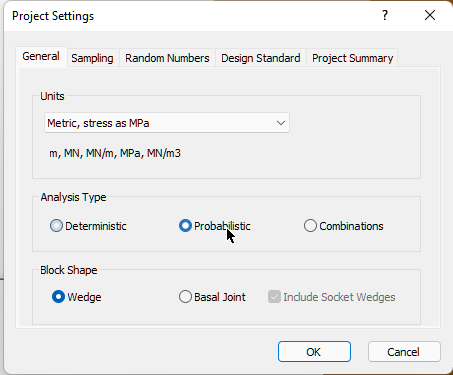1. Select the General tab,
2. Set Analysis Type = Probabilistic.
3. Click OK.

### 4.2 INPUT PROBABILISTIC DATA

Now we'll define the random variables for the Probabilistic analysis. For bolt properties, this is done through the Bolt Properties dialog. To open the dialog, select Bolt Properties on the toolbar of the Support menu.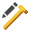Select: Support > Bolt Properties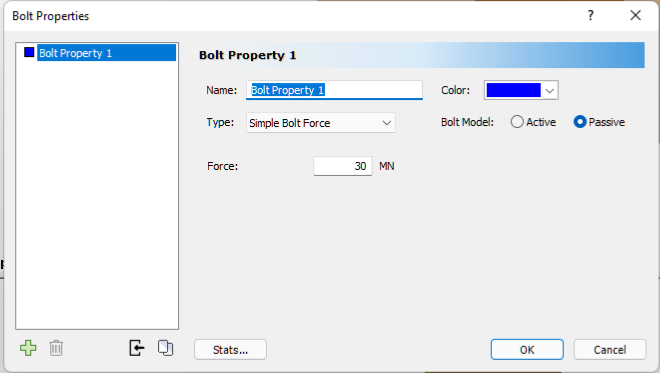1. Click on the Stats button
2. In the Statistics dialog, specify a Normal distribution with a Mean of 30 and Std. Dev. of 10.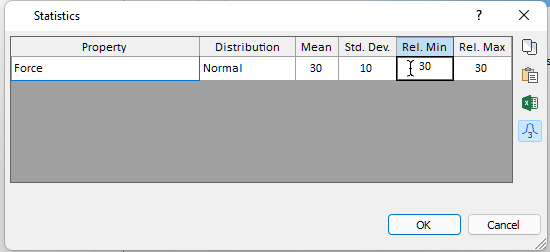The relative min and max would be set to 3x the std. dev., which is 30, by default.

3. Click OK to close the statistics input.
4. Click OK again to exit the bolt properties dialog.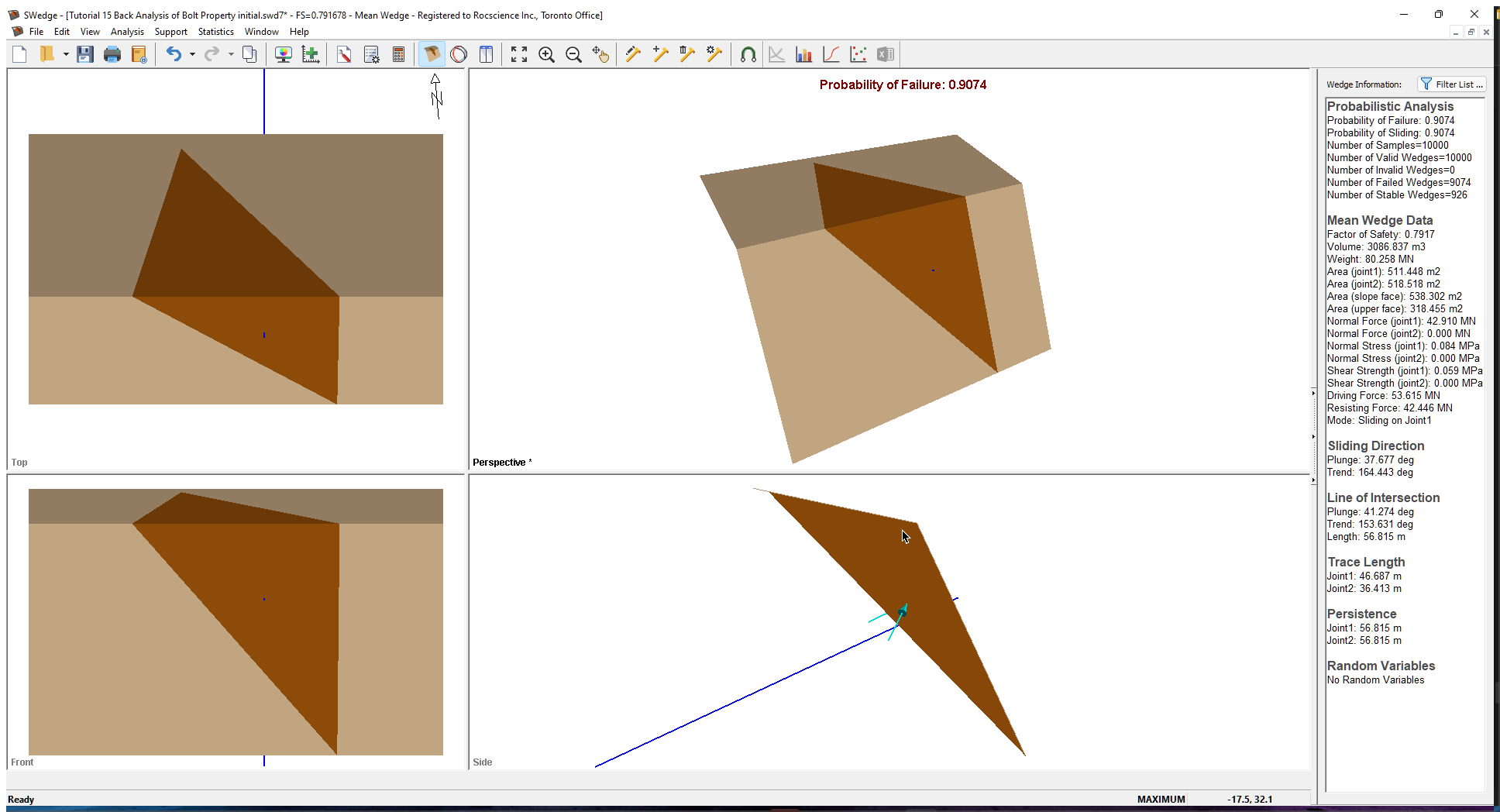The probability of failure is 0.9074.

### 4.3 HISTOGRAMS

Now let's plot of a histogram of the Probabilistic analysis results, using the Histogram Plot Parameters dialog. To open the dialog, select Plot Histogram on the toolbar or Statistics menu.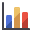Select: Statistics > Plot Histogram

Keep the default settings and click OK.The following plot is generated.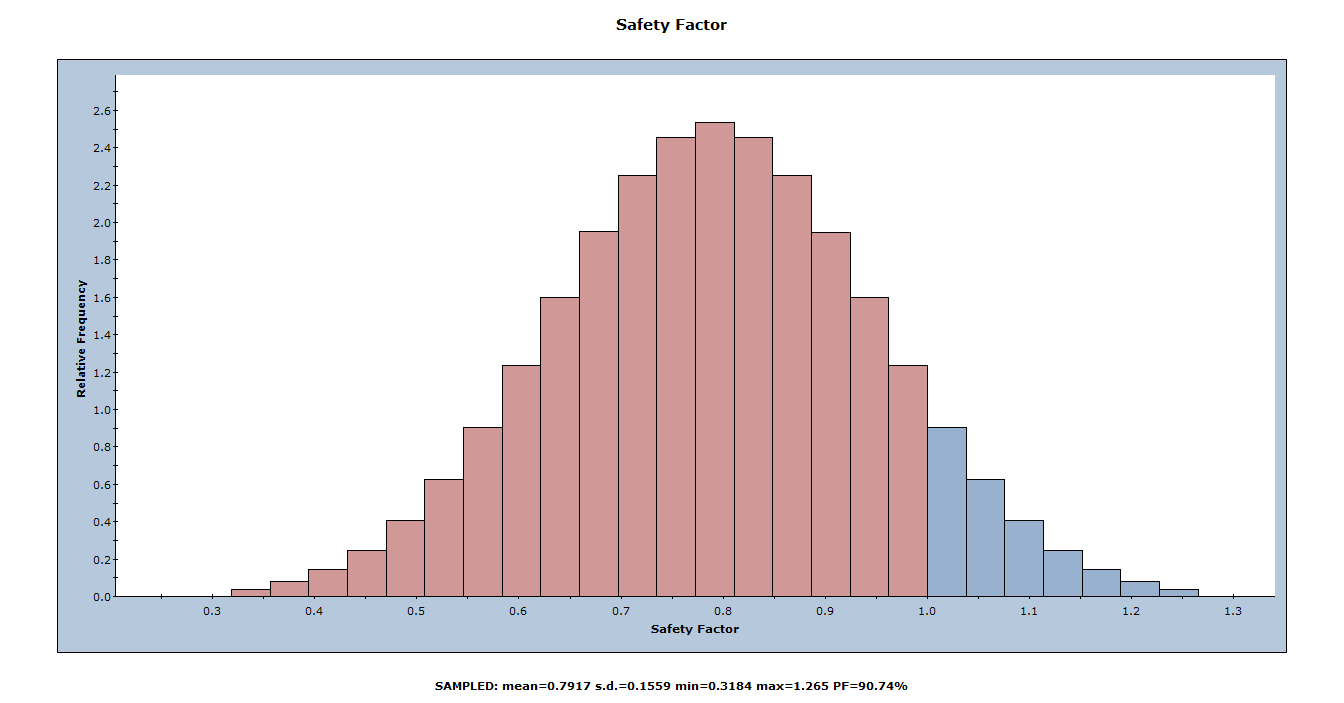The red bars at the left of the distribution represent wedges with a Safety Factor of less than 1.0. We know from the previous sensitivity analysis that a 43.2 MN/m passive bolt force is required to achieve a safety factor of 1.0. With a mean value of only 30 MN/m, over half of the wedges failed. We can also see at the bottom that the factor of safety is only 0.7917 with 30 MN/m bolt force. Let's plot the FoS against the bolt forces with a scatter plot.

### 4.4 SCATTER PLOTS

Scatter plots allow you to examine the relationships between analysis variables. You generate a Scatter plot using the Scatter Plot Parameters dialog.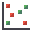Select: Statistics > Plot Scatter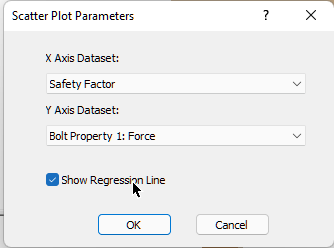1. In the Scatter Plot Parameters dialog, select Safety Factor vs. Bolt Property 1: Force.
2. Select the Show Regression Line check box.
3. Click OK.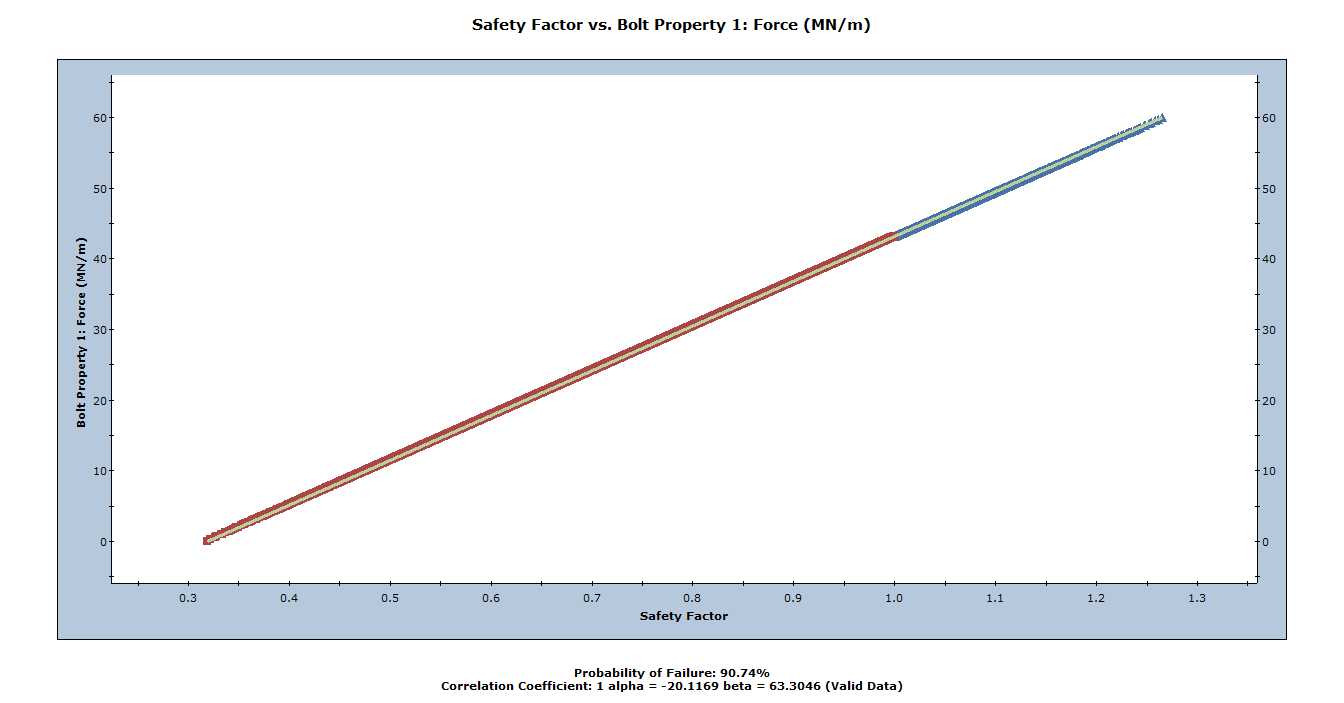The Correlation Coefficient listed at the bottom of the plot indicates the degree of correlation between the two variables plotted. We can see that the FoS and the passive bolt force are related by a linear transformation. That is correct since the only statistical variable in this model is the passive bolt force.

Let's turn on the sampler again and zoom in on where the FoS is 1.0.We see that a value of 43.19 MN/m passive bolt force corresponds to safety factor = 1.0, which matches what we obtained from the sensitivity analysis. The only difference between the 2 analyses is the number of samples. Sensitivity analysis uses 51 samples and the probabilistic analysis uses 10000 samples.

This concludes the back analysis of bolt properties tutorial.